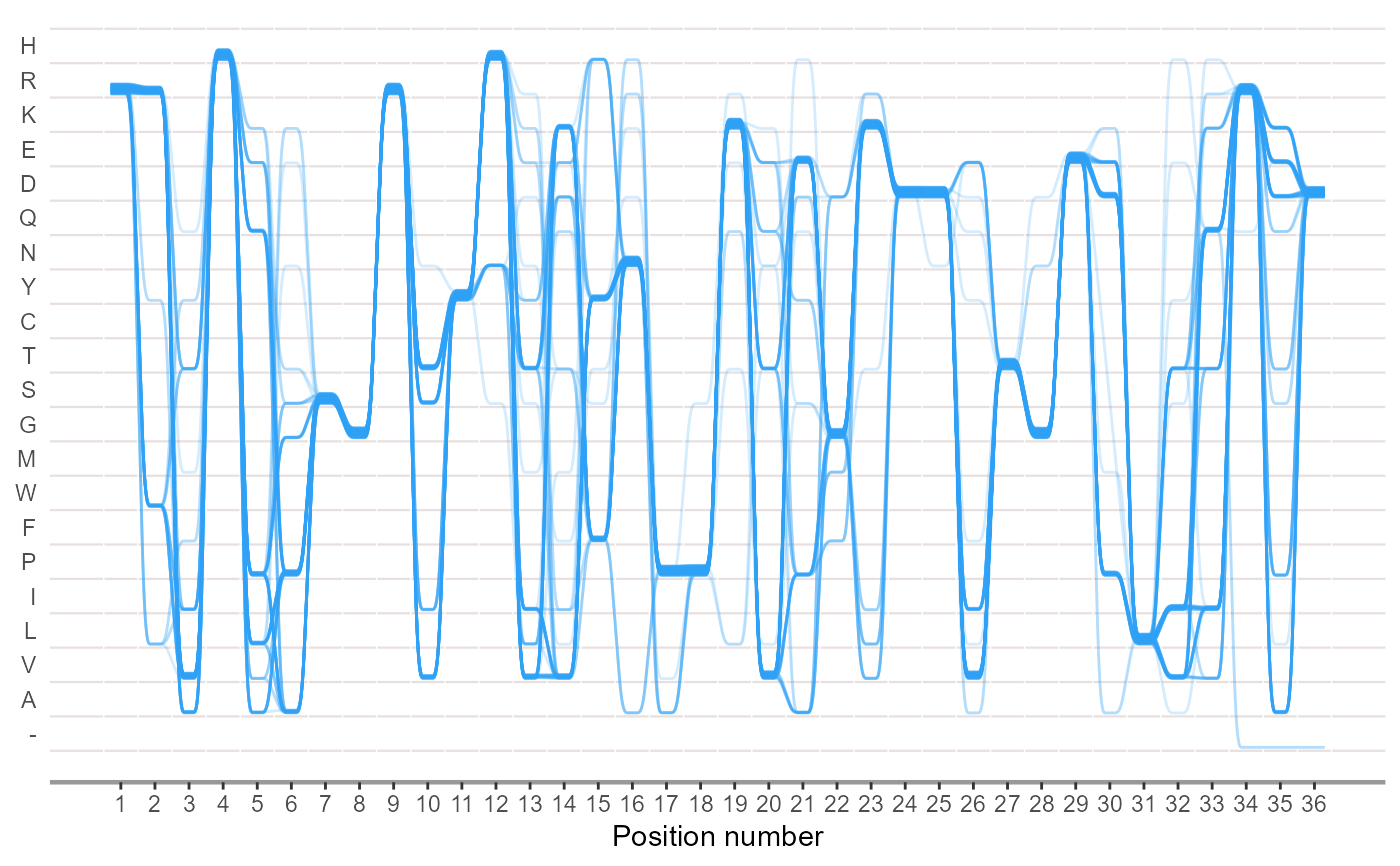plot Sequence Bundles for MSA based 'ggolot2'

ggSeqBundle(
msa,
line_widch = 0.3,
line_thickness = 0.3,
line_high = 0,
spline_shape = 0.3,
size = 0.5,
alpha = 0.2,
bundle_color = c("#2ba0f5", "#424242"),
lev_molecule = c("-", "A", "V", "L", "I", "P", "F", "W", "M", "G", "S", "T", "C",
"Y", "N", "Q", "D", "E", "K", "R", "H")
)

## Arguments

msa Multiple sequence alignment file(FASTA) or object for representing either nucleotide sequences or peptide sequences.Also receives multiple MSA files.eg:msa = c("Gram-negative_AKL.fasta", "Gram-positive_AKL.fasta"). The width of bundles at each site, default is 0.3. The thickness of bundles at each site, default is 0.3. The high of bundles at each site, default is 0. A numeric vector of values between -1 and 1, which control the shape of the spline relative to the control points.From ggalt::geom_xspline(). A numeric vector of values between o and 1, which control the size of each lines. A numeric vector of values between o and 1, which control the alpha of each lines. The colors of each sequence bundles.eg: bundle_color = c("#2ba0f5","#424242"). Reassigning the Y-axis and displaying letter-coded amino acids/nucleotides arranged by physiochemical properties or others.eg:amino acids hydrophobicity lev_molecule = c("-","A", "V", "L", "I", "P", "F", "W", "M", "G", "S","T", "C", "Y", "N", "Q", "D", "E", "K","R", "H").

ggplot object

Lang Zhou

## Examples

aln <- system.file("extdata", "Gram-negative_AKL.fasta", package = "ggmsa")
ggSeqBundle(aln)